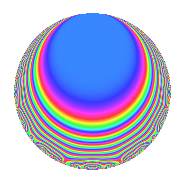# Properties

 Label 500.1.hLevel 500 Weight 1 Character orbit h Rep. character $$\chi_{500}(99,\cdot)$$ Character field $$\Q(\zeta_{10})$$ Dimension 8 Newforms 1 Sturm bound 75 Trace bound 0

# Related objects

## Defining parameters

 Level: $$N$$ = $$500 = 2^{2} \cdot 5^{3}$$ Weight: $$k$$ = $$1$$ Character orbit: $$[\chi]$$ = 500.h (of order $$10$$ and degree $$4$$) Character conductor: $$\operatorname{cond}(\chi)$$ = $$100$$ Character field: $$\Q(\zeta_{10})$$ Newforms: $$1$$ Sturm bound: $$75$$ Trace bound: $$0$$

## Dimensions

The following table gives the dimensions of various subspaces of $$M_{1}(500, [\chi])$$.

Total New Old
Modular forms 48 32 16
Cusp forms 8 8 0
Eisenstein series 40 24 16

The following table gives the dimensions of subspaces with specified projective image type.

$$D_n$$ $$A_4$$ $$S_4$$ $$A_5$$
Dimension 8 0 0 0

## Trace form

 $$8q$$ $$\mathstrut +\mathstrut 2q^{4}$$ $$\mathstrut +\mathstrut 2q^{9}$$ $$\mathstrut +\mathstrut O(q^{10})$$ $$8q$$ $$\mathstrut +\mathstrut 2q^{4}$$ $$\mathstrut +\mathstrut 2q^{9}$$ $$\mathstrut -\mathstrut 2q^{16}$$ $$\mathstrut -\mathstrut 4q^{26}$$ $$\mathstrut +\mathstrut 4q^{29}$$ $$\mathstrut -\mathstrut 6q^{34}$$ $$\mathstrut -\mathstrut 2q^{36}$$ $$\mathstrut -\mathstrut 4q^{41}$$ $$\mathstrut -\mathstrut 8q^{49}$$ $$\mathstrut -\mathstrut 4q^{61}$$ $$\mathstrut +\mathstrut 2q^{64}$$ $$\mathstrut +\mathstrut 4q^{74}$$ $$\mathstrut -\mathstrut 2q^{81}$$ $$\mathstrut -\mathstrut 6q^{89}$$ $$\mathstrut +\mathstrut O(q^{100})$$

## Decomposition of $$S_{1}^{\mathrm{new}}(500, [\chi])$$ into irreducible Hecke orbits

Label Dim. $$A$$ Field Image CM RM Traces $q$-expansion
$$a_2$$ $$a_3$$ $$a_5$$ $$a_7$$
500.1.h.a $$8$$ $$0.250$$ $$\Q(\zeta_{20})$$ $$D_{5}$$ $$\Q(\sqrt{-1})$$ None $$0$$ $$0$$ $$0$$ $$0$$ $$q-\zeta_{20}q^{2}+\zeta_{20}^{2}q^{4}-\zeta_{20}^{3}q^{8}-\zeta_{20}^{4}q^{9}+\cdots$$